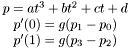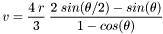# Bezier Lines and Patches

This page is a result of me finding hardly any very useful documentation about cubic tensor product patches and how to subdivide them easily. It is also a convenient place for me to go to in order to re-learn what I keep forgetting. It is a part of the Laxkit documentation.

By Tom Lechner. This page is released under the Creative Commons Attribution Non-Commercial Share-Alike license.

## Rolling With Bezier Lines

Say you want a cubic curve defined by two vertex points (p0 and p3) and two control points (p1 and p2). The tangent at a vertex will be proportional to the vector from the vertex point to the control point. Define the curve so that it is a function of t going from 0 to 1. Then:If we take g=3, then we have the most common type of this sort of cubic curve. So from the above we find:orNote that.

This curve has the convenient subdividable property that with points p0 to p3, we can subdivide this so that we have 2 new segments whose points are [p0 q1 q2 q6] and [q6 q4 q5 p3]. Note also that a bezier segment will always be contained within the bouning box of the vertex and control points. More specifically, the curve will always be contained within the convex hull of those points.## Fun With Bezier Lines

Say you want to approximate a circular arc with a cubic bezier line. For approximation purposes, the t=.5 point of the bezier curve must pass through the middle of the arc. Then for a circle of radius r and arc angle, it follows that the length of the vector connecting a vertex point and its control point is v:## Groking Bezier Patcheswhere p are either the x or y coordinates of the points, depending on whether you want the x or y coordinate of P.

(*** TODO: explain where the hell that patch equation came from)

## How To Subdivide a Cubic Bezier Patch

Have the patch be defined as a funtion ofandthat each run from 0 to 1. You want a section of the patch as a function ofandwhich also run from 0 to 1.

Forand, make corresponding matrices:These will be linearly related toandlike this:So, andwhere:Use those plus the above equation for a patch and get points Q:whereis the plain bezier curve matrix,is the coordinate matrix of the original patch as above, andmagically contains the coordinate matrix of the new patch for u and v.

Generated on Wed Apr 19 20:14:55 2006 for formulatest by1.4.6